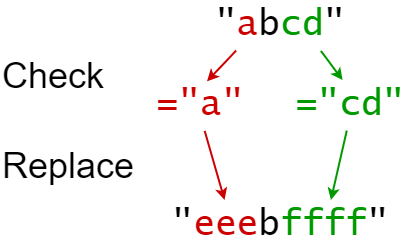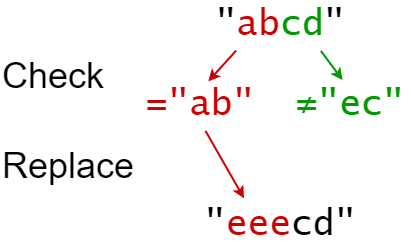GeetCode Hub

You are given a 0-indexed string s that you must perform k replacement operations on. The replacement operations are given as three 0-indexed parallel arrays, indices, sources, and targets, all of length k.

To complete the ith replacement operation:

1. Check if the substring sources[i] occurs at index indices[i] in the original string s.
2. If it does not occur, do nothing.
3. Otherwise if it does occur, replace that substring with targets[i].

For example, if s = "abcd", indices[i] = 0, sources[i] = "ab", and targets[i] = "eee", then the result of this replacement will be "eeecd".

All replacement operations must occur simultaneously, meaning the replacement operations should not affect the indexing of each other. The testcases will be generated such that the replacements will not overlap.

• For example, a testcase with s = "abc", indices = [0, 1], and sources = ["ab","bc"] will not be generated because the "ab" and "bc" replacements overlap.

Return the resulting string after performing all replacement operations on s.

A substring is a contiguous sequence of characters in a string.

Example 1:Input: s = "abcd", indices = [0, 2], sources = ["a", "cd"], targets = ["eee", "ffff"]
Output: "eeebffff"
Explanation:
"a" occurs at index 0 in s, so we replace it with "eee".
"cd" occurs at index 2 in s, so we replace it with "ffff".

Example 2:Input: s = "abcd", indices = [0, 2], sources = ["ab","ec"], targets = ["eee","ffff"]
Output: "eeecd"
Explanation:
"ab" occurs at index 0 in s, so we replace it with "eee".
"ec" does not occur at index 2 in s, so we do nothing.

Constraints:

• 1 <= s.length <= 1000
• k == indices.length == sources.length == targets.length
• 1 <= k <= 100
• 0 <= indexes[i] < s.length
• 1 <= sources[i].length, targets[i].length <= 50
• s consists of only lowercase English letters.
• sources[i] and targets[i] consist of only lowercase English letters.

class Solution { public String findReplaceString(String s, int[] indices, String[] sources, String[] targets) { } }